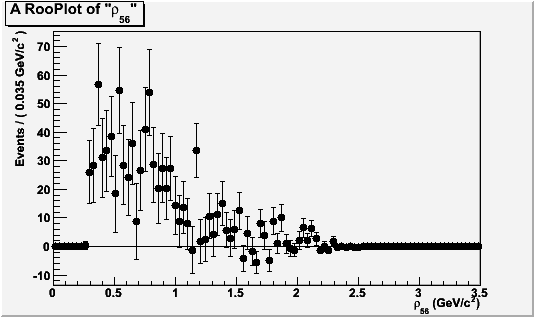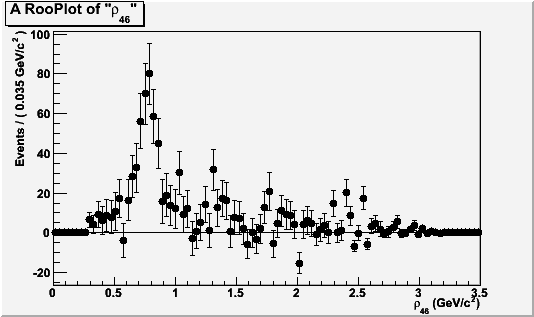# Import dataset

Hi Experts,

I’m doing a simultaneous fit following the example \$ROOTSYS/tutorials/roofit/rf501_simultaneouspdf.C
First of all I save all the results for the “separate fit” in some workspaces and I use two different Pdf to fit them using the same dataset but fitting over 2 different variables.

Then to combine the result in the same fit:

1. Import the weighted dataset twice renaming it in 2 different ways
TFile *fitres = TFile::Open(“file.root”);
RooDataSet rwDataset46 = (RooDataSet) fitres->Get(“dataset_rwLb”);
RooDataSet rwDataset56 = (RooDataSet) fitres->Get(“dataset_rwLb”);
in this way I don’t have problem with “random weigth”

2. Change the name of the 2 variables into the same to be sure to have the same situation of rf501_simultaneouspdf.C
rwDataset46->changeObservableName(“var1”,“var”);
rwDataset56->changeObservableName(“var2”,“var”);
and it works.

3.Define category to distinguish samples events
RooCategory sample(“sample”,“sample”) ;
sample.defineType(“physics”) ;
sample.defineType(“control”) ;

1. Combine the 2 previous datasets in this way:

RooDataSet combData(“combData”,“combined data”,var,Index(sample),Import(“physics”,*rwDataset46),Import(“control”,*rwDataset56)) ;

1. Construct a simultaneous pdf using category sample as index and associate model with the “sample”
RooSimultaneous simPdf(“simPdf”,“simultaneous pdf”,sample) ;

I don’t understand why the combined dataset is the all dataset and no the weigthed one that I want to use.
Plots are posted in the wrong order. See them from bottom to top

ThanksHi,

A problem with the handling of weights in datasets was discovered recently, which very likely also affects your example. ROOT release 5.27/04 (out this week), will have a fix for this.

Wouter

Hi Experts,

I think that function Import doesn’t work so well.
As I told you I have a weigthed dataset and I wanna use it twice and i can do it simply renaming it in this way:

TFile *fitres = TFile::Open(“file.root”);
RooDataSet dataset_rwLb = (RooDataSet) fitres->Get(“dataset_rwLb”);
RooDataSet rwDataset46 = (RooDataSet) fitres->Get(“dataset_rwLb”);
RooDataSet rwDataset56 = (RooDataSet) fitres->Get(“dataset_rwLb”);
and this is the output

++++dataset_rwLb+++++
DataStore data (RooFitSIG)
Contains 23979 entries
Observables:

1. dmfit = 3.11486 L(3.1 - 3.55) // [GeV/c^{2}] “Lb-Lc”
2. mrho2 = 1.26173 L(0 - 3.5) // [GeV/c^{2}] “#rho_{46}”
3. mrho3 = 0.960138 L(0 - 3.5) // [GeV/c^{2}] “#rho_{56}”
4. m3pi = 1.68436 L(0 - 3.5) // [GeV/c^{2}] “m3#pi”
5. L_nLbsig = 3.14021e-23 C L(-INF - +INF) “L_nLbsig”
6. nBkg_sw = 1.17678 C L(-INF - +INF) “nBkg_sw”
7. L_nBkg = 4.06221 C L(-INF - +INF) "L_nBkg"
Dataset variable “nLbsig_sw” is interpreted as the event weight

++++rwDataset46+++++
DataStore data (RooFitSIG)
Contains 23979 entries
Observables:

1. dmfit = 3.11486 L(3.1 - 3.55) // [GeV/c^{2}] “Lb-Lc”
2. mrho2 = 1.26173 L(0 - 3.5) // [GeV/c^{2}] “#rho_{46}”
3. mrho3 = 0.960138 L(0 - 3.5) // [GeV/c^{2}] “#rho_{56}”
4. m3pi = 1.68436 L(0 - 3.5) // [GeV/c^{2}] “m3#pi”
5. L_nLbsig = 3.14021e-23 C L(-INF - +INF) “L_nLbsig”
6. nBkg_sw = 1.17678 C L(-INF - +INF) “nBkg_sw”
7. L_nBkg = 4.06221 C L(-INF - +INF) "L_nBkg"
Dataset variable “nLbsig_sw” is interpreted as the event weight

++++rwDataset56+++++
DataStore data (RooFitSIG)
Contains 23979 entries
Observables:

1. dmfit = 3.11486 L(3.1 - 3.55) // [GeV/c^{2}] “Lb-Lc”
2. mrho2 = 1.26173 L(0 - 3.5) // [GeV/c^{2}] “#rho_{46}”
3. mrho3 = 0.960138 L(0 - 3.5) // [GeV/c^{2}] “#rho_{56}”
4. m3pi = 1.68436 L(0 - 3.5) // [GeV/c^{2}] “m3#pi”
5. L_nLbsig = 3.14021e-23 C L(-INF - +INF) “L_nLbsig”
6. nBkg_sw = 1.17678 C L(-INF - +INF) “nBkg_sw”
7. L_nBkg = 4.06221 C L(-INF - +INF) "L_nBkg"
Dataset variable “nLbsig_sw” is interpreted as the event weight

Then I wanna combine them to do a Simultaneous fit and I do in this way:

RooCategory sample(“sample”,“sample”) ;
sample.defineType(“physics”) ;
sample.defineType(“control”) ;

// Construct combined dataset in (x,sample)
RooDataSet combData(“combData”,“combined data”,Mass_bum,Index(sample),Import(“physics”,*rwDataset46),Import(“control”,*rwDataset56)) ;

but now the output is this:

++++combData+++++

DataStore combData (RooFitSIG)

Contains 40480 entries
Observables:

1. sample = physics “sample”
2. mrho_bum = 1.26173 L(0.5 - 2.5) “#pi#pi Mass [GeV/c^{2}]”

and this is not my dataset.
What’s happening?

Thanks

Hi,

I think you also need to add a WeightVar(“nLbsig_sw”) to the RooDataSet constructor.

Wouter